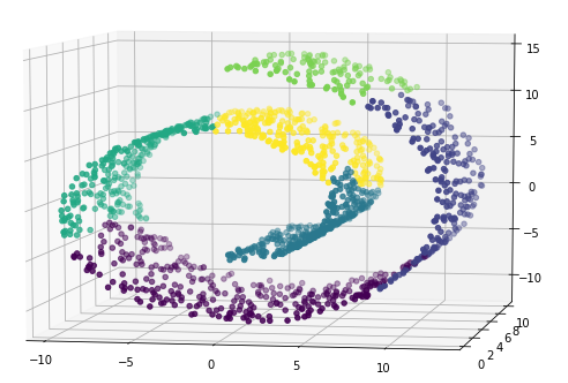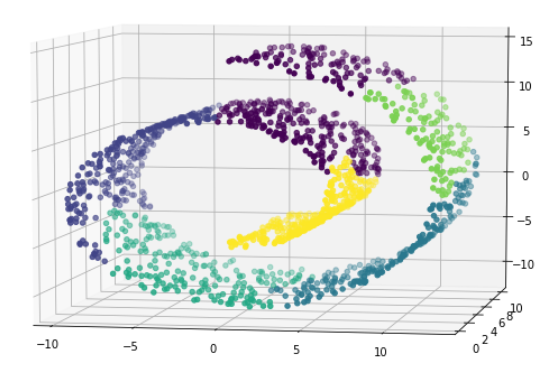## 聚类-分层聚类

1.分层聚类的介绍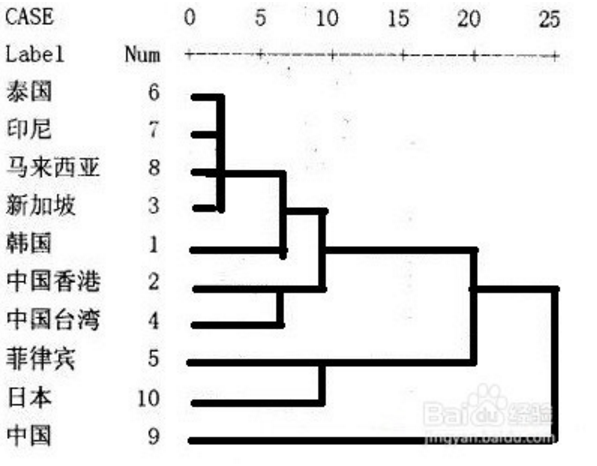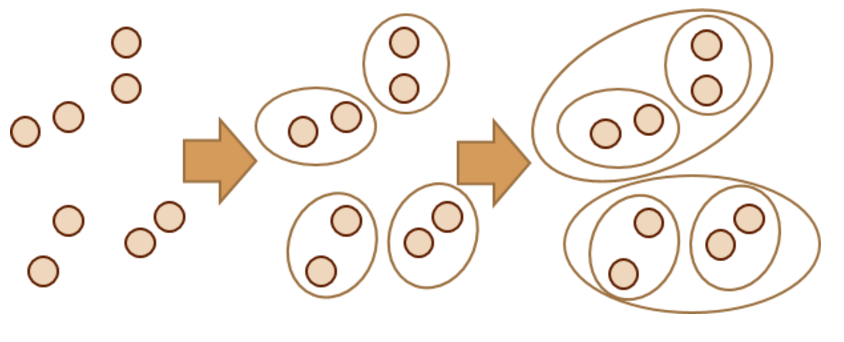2.两个组合数据点之间的距离：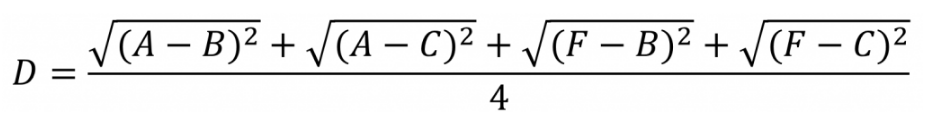http://blog.sciencenet.cn/blog-2827057-921772.html

3.分层聚类的案例

``` 1 import warnings
2 warnings.filterwarnings('ignore')
3 import numpy as np
4 import matplotlib.pyplot as plt
5 %matplotlib inline
6 from sklearn import datasets
7 # 凝聚聚类：自下而上聚类
8 from sklearn.cluster import AgglomerativeClustering,KMeans
9 from mpl_toolkits.mplot3d.axes3d import Axes3D
10
11 # 加载数据
12 X,t = datasets.make_swiss_roll(n_samples=1500,noise = 0.05)
13 # 纵坐标变‘薄’，离的更近
14 X[:,1]*=0.5
15 # (1500, 3) (1500,)三维的
16 display(X.shape,t.shape)
17
18 # 画图
19 fig = plt.figure(figsize=(9,6))
20 axes3D = Axes3D(fig)
21 axes3D.scatter(X[:,0],X[:,1],X[:,2],c =t)
22 # 调整角度
23 axes3D.view_init(7,-80)```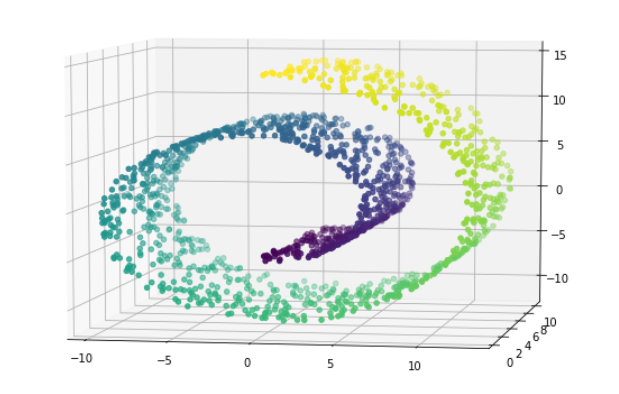```1 # 使用kmeans
2 kmeans = KMeans(6)
3 kmeans.fit(X)
4 y_ = kmeans.predict(X)
5
6 fig = plt.figure(figsize=(9,6))
7 axes3D = Axes3D(fig)
8 axes3D.scatter(X[:,0],X[:,1],X[:,2],c = y_)
9 axes3D.view_init(7,-80)```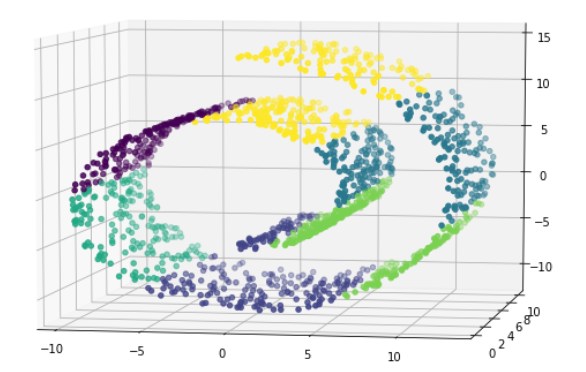kmeans效果不好，和上图比较一下就好

``` 1 from sklearn.neighbors import kneighbors_graph
2
3 # linkage : {"ward", "complete", "average", "single"}
4 conn = kneighbors_graph(X,5)
6
7 agg.fit(X)
8
9 y_ = agg.labels_
10
11 fig = plt.figure(figsize=(9,6))
12 axes3D = Axes3D(fig)
13
14 axes3D.scatter(X[:,0],X[:,1],X[:,2],c = y_)
15
16 axes3D.view_init(7,-80)```